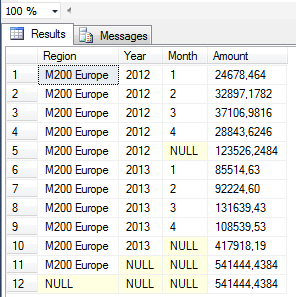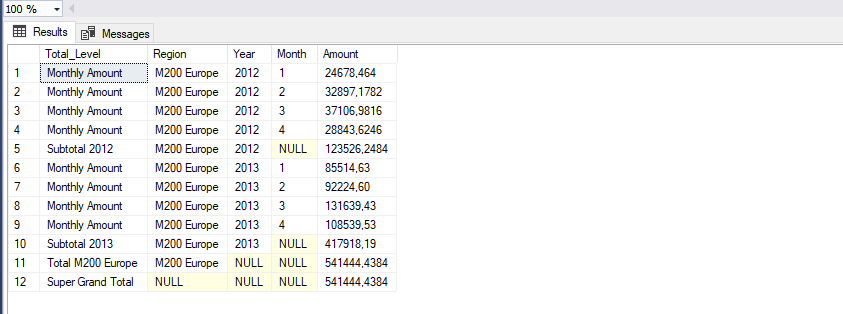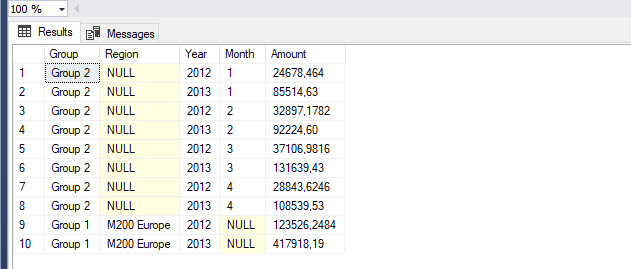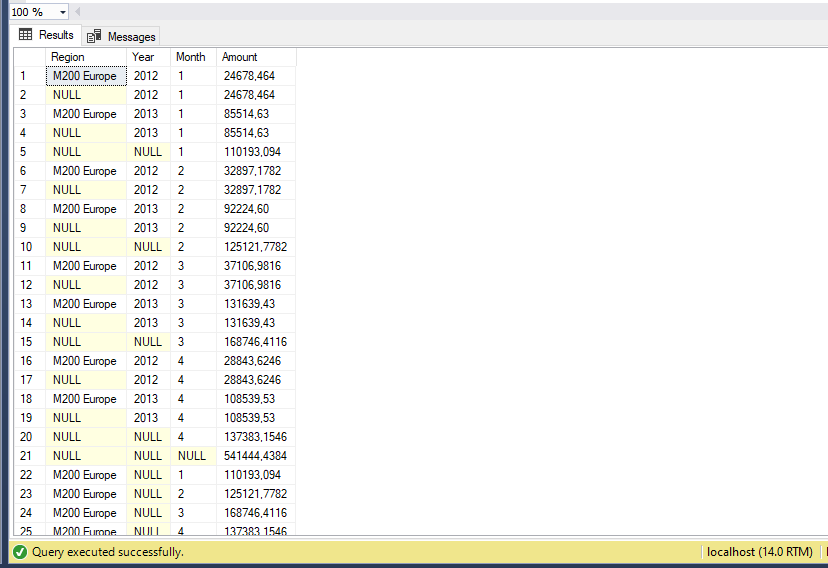• 15. 2. 2020
• Jan Zedníček - Data & Finance
• 0

What about upgrading GROUP BY clause by use of useful commands? GROUP BY is used in a clause with aggregate operations. When it is used, aggregation happens throughout all columns. Using this simple “grouping” does not enable us to do totals and subtotals. But there is plenty of operators which can easily query for totals.

After you get to know these operators, you will be able to create SQL queries and views with total rows. That is not something anyone could do. 🙂 All three operators work in a similar manner. So I will do ROLLUP  in detail and the rest will be just fast flyby.

## 1) ROLLUP | CUBE | GROUPING SETS Syntax

`SELECT [Column1], [Column2], AggregateFunction([Column3]) AS Alias`
` FROM Table`
` WHERE Condition`
` GROUP BY ROLLUP ([Column1], [Column2]) |or| CUBE ([Column1], [Column2]) |or| GROUPING SETS ([Column1], [Column2]);`

## 2) Totals and Subtotals Using Columns Through All Levels – ROLLUP

First operator is ROLLUP. It returns totals throughout all levels (columns). The most effective demonstration will be on example where we will display sales in first 4 months of year 2012 and 2013 in Europe region.

`SELECT`
`  [ModelRegion]      AS [Region],`
`  [CalendarYear]     AS [Year],`
`  [Month]            AS [Month],`
`  SUM([Amount])      AS [Amount]`
` FROM [AdventureWorksDW2014].[dbo].[vTimeSeries]`
` WHERE [CalendarYear] in (2012,2013) AND [month]<=4 AND [ModelRegion] in ('M200 Europe')`
` GROUP BY ROLLUP([ModelRegion], [CalendarYear], [Month]);`

### Result After ROLLUP Application

We can see that the query returns 4 rows more and contains all subtotals and total thanks to ROLLUP.The result can be further upgraded by function which is useful mainly when working with ROLLUP and similar operators. It is function GROUPING(). It accepts name of the column as parameter.

Function returns 1 or 0 depending if the given column is Total/subtotal or original base value. I will show it to you on the previous example by editing SQL query.

` SELECT`
`  CASE`
`    WHEN GROUPING([ModelRegion]) = 1 THEN 'Super Grand Total'`
`    WHEN GROUPING([CalendarYear]) = 1 THEN (CONCAT('Total ',[ModelRegion]))`
`    WHEN GROUPING([Month]) = 1 THEN CONCAT('Subtotal ',[CalendarYear])`
`    ELSE 'Monthly Amount'`
`  END AS [Total_Level],`
`  [ModelRegion]       AS [Region],`
`  [CalendarYear]      AS [Year],`
`  [Month]             AS [Month],`
`  SUM([Amount])       AS [Amount]`
`FROM [AdventureWorksDW2014].[dbo].[vTimeSeries]`
`WHERE [CalendarYear] in (2012,2013) AND [month]<=4 AND [ModelRegion] in ('M200 Europe')`
`GROUP BY ROLLUP (`
`  [ModelRegion]`
`  ,[CalendarYear]`
`  ,[Month]`
`);`We marked each row by flag using the function depending on type of total.

Item Super grand total is in this case equal to total count of Europe region since we did not involve any other region. If we would have more regions, the total would be a total of the regions.

## 3) GROUPING SETS  – Create Your Own Aggregate Groups

You can create your own groups through which the aggregate operations will perform. Multiple groups may be defined. I will create 2 aggregate groups on the example below

• Subtotals through region and year
• Subtotals through year and month

` SELECT`
`  CASE`
`    WHEN [ModelRegion] IS NULL THEN 'Group 2'`
`    ELSE 'Group 1'`
`  END AS [Group],`
`  [ModelRegion]       AS [Region],`
`  [CalendarYear]      AS [Year],`
`  [Month]             AS [Month],`
`  SUM([Amount])       AS [Amount]`
`FROM [AdventureWorksDW2014].[dbo].[vTimeSeries]`
`WHERE [CalendarYear] in (2012,2013) AND [month]<=4 AND [ModelRegion] in ('M200 Europe')`
`GROUP BY GROUPING SETS (`
`    ([ModelRegion],[CalendarYear]),`
`    ([CalendarYear],[Month])`
`); `## 4) CUBE – Cube of Totals

This command creates totals throughout all value combinations in columns indexed as argument. To identify totals and subtotals we can use GROUPING function mentioned earlier

` SELECT`
`   [ModelRegion]       AS [Region],`
`   [CalendarYear]      AS [Year],`
`   [Month]             AS [Month],`
`   SUM([Amount])       AS [Amount]`
`FROM [AdventureWorksDW2014].[dbo].[vTimeSeries]`
`WHERE [CalendarYear] in (2012,2013) AND [month]<=4 AND [ModelRegion] in ('M200 Europe')`
`GROUP BY CUBE (`
`  [ModelRegion]`
`  ,[CalendarYear]`
`  ,[Month]`
`); `Rate this post### Jan Zedníček - Data & Finance

My name is Jan Zedníček and I work as a freelancer. I have been working as a freelancer for many companies for more than 10 years.
I used to work as a financial controller, analyst and manager at many different companies in field of banking and manufacturing. When I am not at work, I like playing volleyball, chess, doing a workout in the gym and I enjoy tasting of best quality rums.
I am trying to summarize all my knowledge on this website not to forget them and to put them forward to anyone.